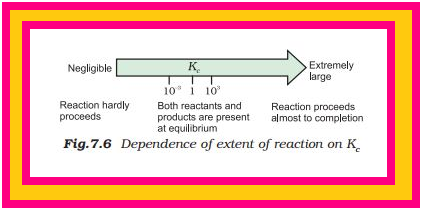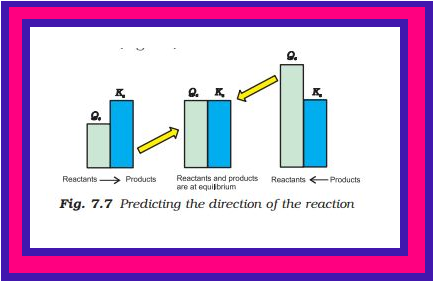Chemistry EQUILIBRIUM CONSTANT

### Topic to be covered

star APPLICATIONS OF EQUILIBRIUM CONSTANTS.
star Predicting the Extent of a Reaction.
star Predicting the Direction of the Reaction.
star Calculating Equilibrium Concentrations.

### APPLICATIONS OF EQUILIBRIUM CONSTANTS

Before considering the applications of equilibrium constants, let us summarise the important features of equilibrium constants as follows:

1. Expression for equilibrium constant is applicable only when concentrations of the reactants and products have attained constant value at equilibrium state.

2. The value of equilibrium constant is independent of initial concentrations of the reactants and products.

3. Equilibrium constant is temperature dependent having one unique value for a particular reaction represented by a balanced equation at a given temperature.

4. The equilibrium constant for the reverse reaction is equal to the inverse of the equilibrium constant for the forward reaction.

5. The equilibrium constant color{red}(K) for a reaction is related to the equilibrium constant of the corresponding reaction, whose equation is obtained by multiplying or dividing the equation for the original reaction by a small integer.

Let us consider applications of equilibrium constant to:

• predict the extent of a reaction on the basis of its magnitude,

• predict the direction of the reaction, and

• calculate equilibrium concentrations.

### Predicting the Extent of a Reaction=> The numerical value of the equilibrium constant for a reaction indicates the extent of the reaction.

=> But it is important to note that an equilibrium constant does not give any information about the rate at which the equilibrium is reached.

=> The magnitude of color{red}(K_c) or color{red}(K_p) is directly proportional to the concentrations of products (as these appear in the numerator of equilibrium constant expression) and inversely proportional to the concentrations of the reactants (these appear in the denominator). This implies that a high value of color{red}(K) is suggestive of a high concentration of products and vice-versa.

We can make the following generalisations concerning the composition of equilibrium mixtures:

• If color{red}(K_c > 10^3), products predominate over reactants, i.e., if color{red}(K_c) is very large, the reaction proceeds nearly to completion. Consider
the following examples:

(a) The reaction of color{red}(H_2) with color{red}(O_2) at 500 K has a very large equilibrium constant , color{red}(K_c = 2.4 × 10^(47)).

(b) color{red}(H_2(g) + Cl_2(g) ⇌ 2HCl(g)) at 300K has color{red}(K_c = 4.0 × 10^(31)).

(c) color{red}(H_2(g) + Br_2(g) ⇌ 2HBr (g)) at 300 K, color{red}(K_c = 5.4 × 10^(18))

• If color{red}(K_c < 10^(–3)), reactants predominate over products, i.e., if color{red}(K_c) is very small, the reaction proceeds rarely. Consider the following examples:

(a) The decomposition of color{red}(H_2O) into color{red}(H_2) and color{red}(O_2) at 500 K has a very small equilibrium constant, color{red}(K_c = 4.1 × 10^(–48))

(b) color{red}(N_2(g) + O_2(g) ⇌ 2NO(g)), at 298 K has color{red}(K_c = 4.8 ×10^(–31)).

• If color{red}(K_c) is in the range of color{red}(10^(–3)) to color{red}(10^3), appreciable concentrations of both reactants and products are present. Consider the following examples:

(a) For reaction of color{red}(H_2) with color{red}(I_2) to give color{red}(HI, K_c = 57.0) at 700K.

(b) Also, gas phase decomposition of color{red}(N_2O_4) to color{red}(NO_2) is another reaction with a value of color{red}(K_c = 4.64 × 10^(–3)) at 25°C which is neither too small nor too large. Hence, equilibrium mixtures contain appreciable concentrations of both color{red}(N_2O_4) and color{red}(NO_2).

These generarlisations are illustrated in Fig. 7.6

### Predicting the Direction of the Reaction=> The equilibrium constant helps in predicting the direction in which a given reaction will proceed at any stage.

=> For this purpose, we calculate the reaction quotient color{red}(Q). The reaction quotient, color{red}(Q) (color{red}(Q_c) with molar concentrations and color{red}(Q_P) with partial pressures) is defined in the same way as the equilibrium constant color{red}(K_c) except that the concentrations in color{red}(Q_c) are not necessarily equilibrium values. For a general reaction:

color{red}(a A + b B ⇌ c C + d D) ........(7.19)

color{red}(Q_c = [C]^c [D]^d // [A]^a [B]^b) ......(7.20)

Then, If color{red}(Q_c > K_c), the reaction will proceed in the direction of reactants (reverse reaction). If color{red}(Q_c < K_c), the reaction will proceed in the
direction of the products (forward reaction).

If color{red}(Q_c = K_c), the reaction mixture is already at equilibrium.

=> Consider the gaseous reaction of color{red}(H_2) with color{red}(I_2),
color{red}(H_2(g) + I_2(g) ⇌ 2HI(g); K_c = 57.0) at 700 K.

=> Suppose we have molar concentrations color{red}([H_2]t=0.10M, [I2]t = 0.20 M) and color{red}([HI]t = 0.40 M). (the subscript color{red}(t) on the concentration symbols means that the concentrations were measured at some arbitrary time color{red}(t), not necessarily at equilibrium).

Thus, the reaction quotient, color{red}(Q_c) at this stage of the reaction is given by,

color{red}(Q_c = [HI]_t^(2) // [H_2]_t [I_2]_t = (0.40)^2// (0.10)×(0.20))

 color{red}(= 8.0)

Now, in this case, color{red}(Q_c (8.0)) does not equal color{red}(K_c (57.0)), so the mixture of color{red}(H_2(g), I_2(g)) and color{red}(HI(g)) is not at equilibrium; that is, more color{red}(H_2(g)) and color{red}(I_2(g)) will react to form more color{red}(HI(g)) and their concentrations will decrease till color{red}(Q_c = K_c)

=> The reaction quotient, color{red}(Q_c) is useful in predicting the direction of reaction by comparing the values of color{red}(Q_c) and color{red}(K_c).

=> Thus, we can make the following generalisations concerning the direction of the reaction (Fig. 7.7)

• If color{red}(Q_c < K_c), net reaction goes from left to right
• If color{red}(Q_c > K_c), net reaction goes from right to left.
• If color{red}(Q_c = K_c), no net reaction occurs.

Q 3039391212The value of Kc for the reaction 2A ⇌ B + C is 2 × 10^(–3). At a given time, the composition of reaction mixture is
[A] = [B] = [C] = 3 × 10^(–4) M. In which direction the reaction will proceed?Solution:

For the reaction the reaction quotient Q_c is given by, Q_c = [B][C]// [A]^2
as [A] = [B] = [C] = 3 × 10^(– 4)M
Q_c = (3 ×10^(–4))(3 × 10^(–4)) / (3 ×10^(–4))^2 = 1
as Q_c > K_c so the reaction will proceed in the reverse direction.

### Calculating Equilibrium Concentrations

=> In case of a problem in which we know the initial concentrations but do not know any of the equilibrium concentrations, the following three steps shall be followed:

Step 1. Write the balanced equation for the reaction.
Step 2. Under the balanced equation, make a table that lists for each substance involved in the reaction:

(a) the initial concentration,
(b) the change in concentration on going to equilibrium, and
(c) the equilibrium concentration. In constructing the table, define color{red}(x) as the concentration (mol/L) of one of the substances
that reacts on going to equilibrium, then use the stoichiometry of the reaction to determine the concentrations of the other substances in terms of color{red}(x).

Step 3. Substitute the equilibrium concentrations into the equilibrium equation for the reaction and solve for color{red}(x). If you are to solve a quadratic equation choose the mathematical solution that makes chemical sense.

Step 4. Calculate the equilibrium concentrations from the calculated value of color{red}(x).

Step 5. Check your results by substituting them into the equilibrium equation.
Q 305959141413.8g of N_2O_4 was placed in a 1L reaction vessel at 400K and allowed to attain equilibrium N_2O_4 (g) ⇌ 2NO_2 (g) The total pressure at equilbrium was found to be 9.15 bar. Calculate K_c, K_p and partial pressure at equilibrium.Solution:

We know pV = nRT
Total volume (V ) = 1 L
Molecular mass of N_2O_4 = 92 g
Number of moles = (13.8g)/(92 g) = 0.15
of the gas (n) Gas constant (R) = 0.083 bar L mol^(–1)K^(–1)
Temperature (T ) = 400 K
pV = nRT
p × 1L = 0.15 mol × 0.083 bar L mol^(–1)K^(–1) × 400 K
p = 4.98 bar

tt(( , N_2O_4 , ⇌, 2NO_2) , ("Initial pressure: ", 4.98 "bar" , ,0) ,("At equilibrium:" , (4.98 – x) "bar" , , 2x "bar"))

Hence, p_text(total) at equilibrium = p_(N_2O_4) +p_(NO_2)
9.15 = (4.98 – x) + 2x
9.15 = 4.98 + x
x = 9.15 – 4.98 = 4.17 bar
Partial pressures at equilibrium are,
p_(N_2O_4) = 4.98 – 4.17 = 0.81 bar
p_(NO_2 ) = 2x = 2 × 4.17 = 8.34 bar

K_p = ( p_(NO_2))^2/p_(N_2O_4)

= (8.34)^2/0.81 = 85.87

K_p = K_c//(RT)^(Delta n)

85.87 = K_c ( 0.083xx400)^1

K_c = 2.586 = 2.6
Q 30896915173.00 mol of PCl_5 kept in 1L closed reaction vessel was allowed to attain equilibrium at 380K. Calculate composition of the
mixture at equilibrium. K_c= 1.80Solution:

tt (( , PCl_5 , ⇌ , PCl_3 , + , Cl_2) , ( text(Initial) , , , , , ) , ("concentration : " , 3.0 , , 0 , , 0) , ("Let x mol per litre of" PCl_5 "be dissociated At equilibrium : " , , , , , ), ( , (3-x) , , x , , x))

K_c = [PCl_3] [Cl_2]//[PCl_5]

1.8 = x^2/(3-x)

x^2 + 1.8x – 5.4 = 0
x = [–1.8 ± √(1.8)2 – 4(–5.4)]/2
x = [–1.8 ± √3.24 + 21.6]/2
x = [–1.8 ± 4.98]/2
x = [–1.8 + 4.98]/2 = 1.59
[PCl_5] = 3.0 – x = 3 –1.59 = 1.41 M
[PCl_3] = [Cl_2] = x = 1.59 M